Courses

# Solid Mechanics- 2

## 10 Questions MCQ Test GATE Civil Engineering (CE) 2022 Mock Test Series | Solid Mechanics- 2

Description
This mock test of Solid Mechanics- 2 for Civil Engineering (CE) helps you for every Civil Engineering (CE) entrance exam. This contains 10 Multiple Choice Questions for Civil Engineering (CE) Solid Mechanics- 2 (mcq) to study with solutions a complete question bank. The solved questions answers in this Solid Mechanics- 2 quiz give you a good mix of easy questions and tough questions. Civil Engineering (CE) students definitely take this Solid Mechanics- 2 exercise for a better result in the exam. You can find other Solid Mechanics- 2 extra questions, long questions & short questions for Civil Engineering (CE) on EduRev as well by searching above.
QUESTION: 1

### The shear stress at the centre of a circular shaft under torsion is

Solution:

The simple torsion equation is written as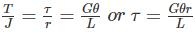This states that the shearing stress varies directly as the distance ‘r' from the axis of the shaft and the following is the stress distribution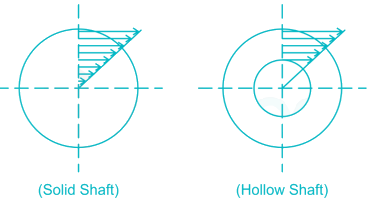Hence the maximum shear stress occurs on the outer surface of the shaft where r = R and at the centre the shear stress is zero.

QUESTION: 2

### Match List-I with List-II and select the correct answer using the code given below the lists. List-I (Loaded Beam) A.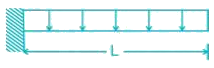B.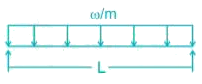C.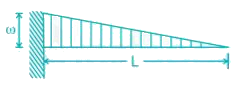List-II (Maximum Bending moment) 1.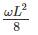2.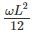3.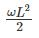4.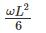Solution:

A-3, B-1, C-4

Beam A is cantilever so maximum bending moment occurs at the fixed support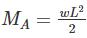Beam B is simply supported so maximum bending moment occurs at midspan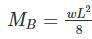Beam C is cantilever so maximum bending moment occurs at fixed support
MC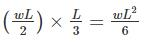QUESTION: 3

### A solid circular shaft of diameter d is subjected to a combined effect of bending moment M and torque T. The relation is used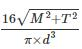for designing the shaft using which one of this material property

Solution: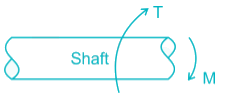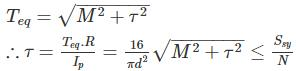Ssy = Torsional yield strength

QUESTION: 4

A solid steel shaft is surrounded by a copper shaft, such that, Isteel = 1/2 Icopper. If Gcopper = 1/2 Gsteel, what is the ratio of Ts/Tc if the composite shaft is subjected to a twisting moment?

Solution: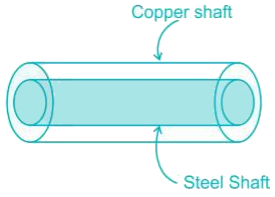Both shaft will rotate through same degree.

Hence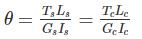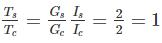QUESTION: 5

Couple M is applied at C on a simply supported beam AB. The maximum shear force on AC will be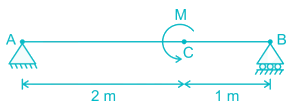Solution: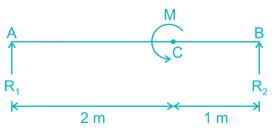R1 + R2 = 0

∑MA = 0

⇒ R2 × 3 = -M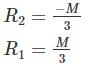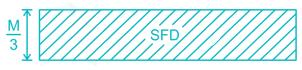So maximum shear force = M/3

QUESTION: 6

A hollow steel shaft of external diameter 100 mm and internal diameter 50 mm is to be replaced by a solid allow shaft. Assuming the same value of polar modulus for both, the diameter of the solid allows shaft will be-

Solution:

Polar modulus,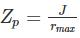Let the diameter of solid shaft is ‘d’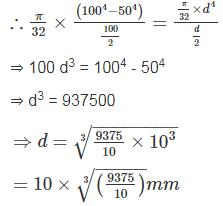*Answer can only contain numeric values
QUESTION: 7

For a loaded cantilever beam of uniform cross-section, the bending moment (in N-mm) along the length is M(x) = 5x2 + 10x, where x is the distance (in mm) measured from the free end of the beam. The magnitude of shear force (in N) in the cross-section at x = 10 mm is ________.

Solution:

M = 5x2 + 10x

Shear Force = dM/dx = 10x + 10

Shear force at X = 10 mm

= 10 x 10 + 10 = 110 N

QUESTION: 8

S.F.D for the beam is: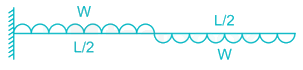Solution: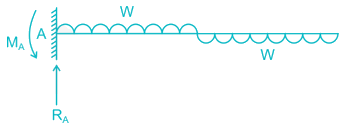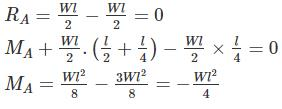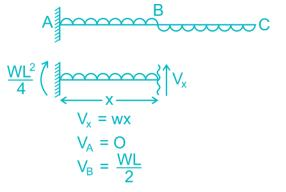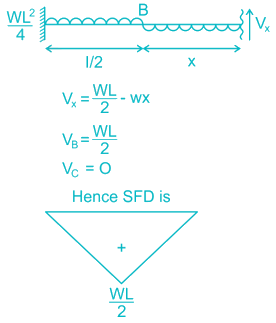QUESTION: 9

The equation for the deflected shape of a beam carrying a U.D.L and simply supported at ends is given below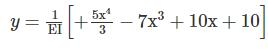The load carrying capacity of the beam is

Solution: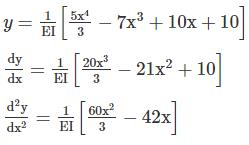Using the equation of deflection: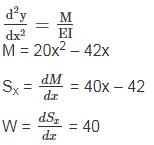W = 40 kN/m

*Answer can only contain numeric values
QUESTION: 10

For the given figure, calculate the bending moment (in kN-m) at 2 m from the left end?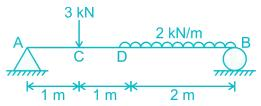Solution: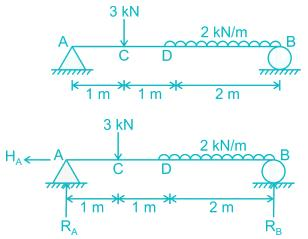∑FV = 0

RA + RB = 3 + 2 × 2

RA + RB = 7 kN

∑FH = 0

HA = 0

∑Mz = 0

RA × 4 – 3 × 3 - 2 × 2 × 1= 0

RA = 3.25 kN

RB = 3.75 kN

BMD = R× 2 - 3 × 1

BMD = 3.25 × 2 - 3 = 3.5 kN